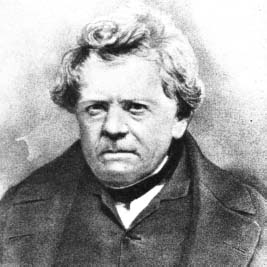# What is Ohm’s Law?

Ohm’s Law is important to the field of electrical studies. It states that direct current flowing in a conductor is directly proportional to the potential difference between its ends. First summarized by German physicist Georg Simon Ohm (1789–1854), it is usually seen in formula form as: V = IR (or I = V/R), in which V is the potential difference (voltage), I is the current (also written in some texts as i), and R is the resistance of the conductor. This can also be written in terms of electric quantities (voltage = current × resistance) and with units of measure (volts = amps × ohms).Georg Ohm discovered the law about how electrical currents flow through a conductor.

Close

This is a web preview of the "The Handy Math Answer Book" app. Many features only work on your mobile device. If you like what you see, we hope you will consider buying. Get the App